# Chapter 25 Electric Current and Direct Current Circuits

• Slides: 40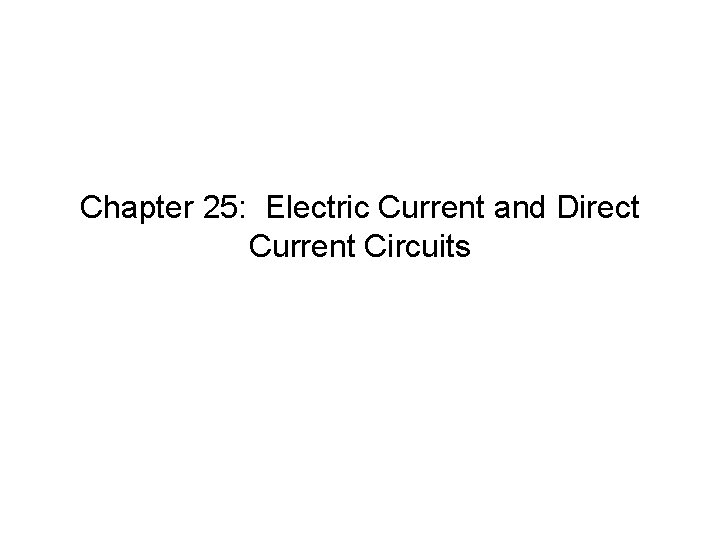Chapter 25: Electric Current and Direct Current CircuitsIf 4. 7 ´ 1016 electrons pass a particular point in a wire every second, what is the current in the wire? a. 4. 7 m. A b. 7. 5 A c. 2. 9 A d. 7. 5 m. A e. 0. 29 AIf 4. 7 ´ 1016 electrons pass a particular point in a wire every second, what is the current in the wire? a. 4. 7 m. A b. 7. 5 A c. 2. 9 A d. 7. 5 m. A e. 0. 29 AThe graph shows the potential difference across a resistor as a function of the current through the resistor. The slope of the resulting curve represents a. power. b. resistance. c. emf. d. charge. e. work per unit charge.The graph shows the potential difference across a resistor as a function of the current through the resistor. The slope of the resulting curve represents a. power. b. resistance. c. emf. d. charge. e. work per unit charge.A resistor carries a current I. The power dissipated in the resistor is P. What is the power dissipated if the same resistor carries current 3 I? a. P b. 3 P c. P/3 d. 9 P e. P/9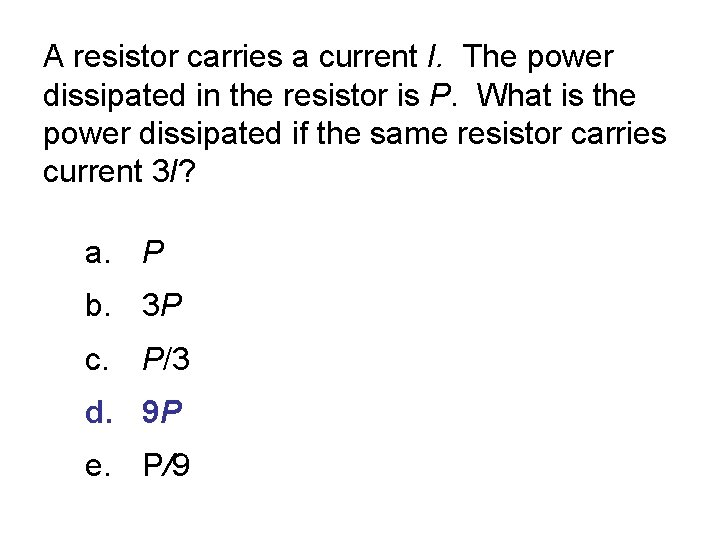A resistor carries a current I. The power dissipated in the resistor is P. What is the power dissipated if the same resistor carries current 3 I? a. P b. 3 P c. P/3 d. 9 P e. P/9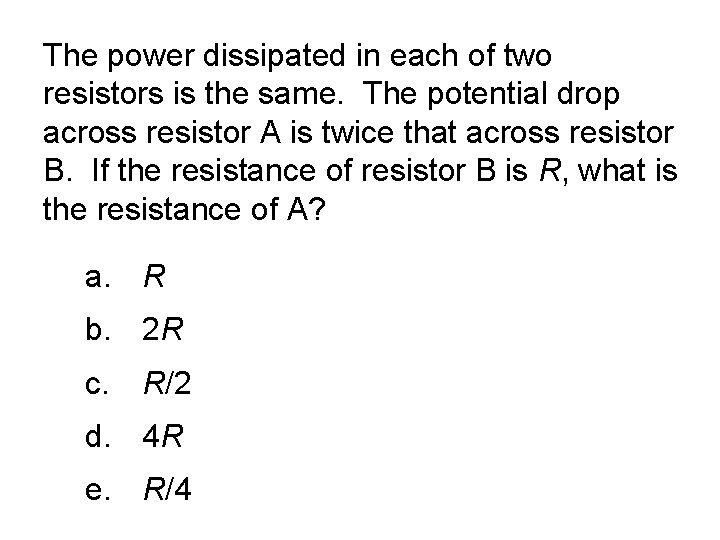The power dissipated in each of two resistors is the same. The potential drop across resistor A is twice that across resistor B. If the resistance of resistor B is R, what is the resistance of A? a. R b. 2 R c. R/2 d. 4 R e. R/4The power dissipated in each of two resistors is the same. The potential drop across resistor A is twice that across resistor B. If the resistance of resistor B is R, what is the resistance of A? a. R b. 2 R c. R/2 d. 4 R e. R/4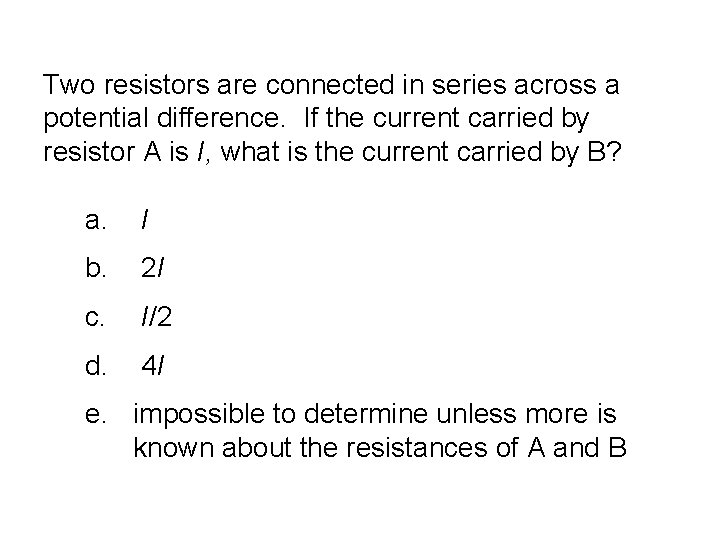Two resistors are connected in series across a potential difference. If the current carried by resistor A is I, what is the current carried by B? a. I b. 2 I c. I/2 d. 4 I e. impossible to determine unless more is known about the resistances of A and B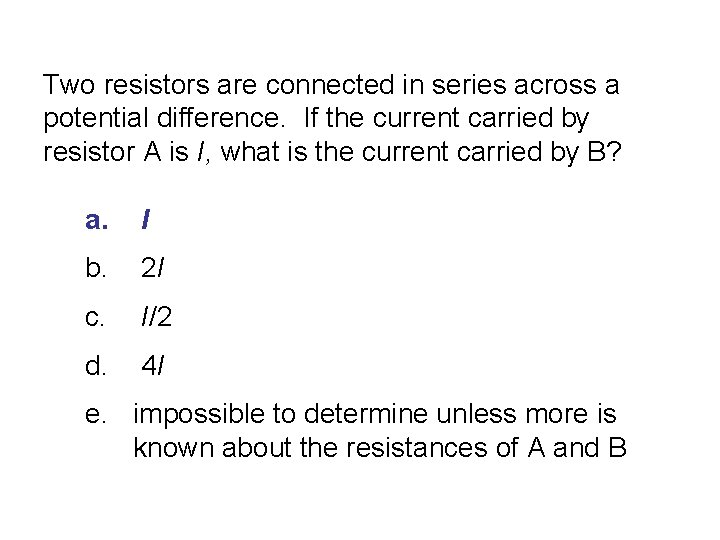Two resistors are connected in series across a potential difference. If the current carried by resistor A is I, what is the current carried by B? a. I b. 2 I c. I/2 d. 4 I e. impossible to determine unless more is known about the resistances of A and BFour identical light bulbs are connected to a power supply as shown. Which light bulb consumes the most power? a. B 1 b. B 2 c. B 3 d. B 4 e. They all consume the same amount of power.Four identical light bulbs are connected to a power supply as shown. Which light bulb consumes the most power? a. B 1 b. B 2 c. B 3 d. B 4 e. They all consume the same amount of power.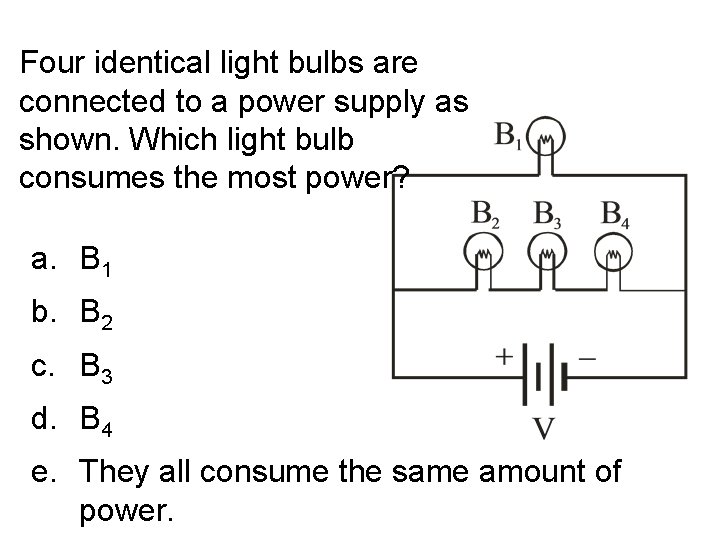Four identical light bulbs are connected to a power supply as shown. Which light bulb consumes the most power? a. B 1 b. B 2 c. B 3 d. B 4 e. They all consume the same amount of power.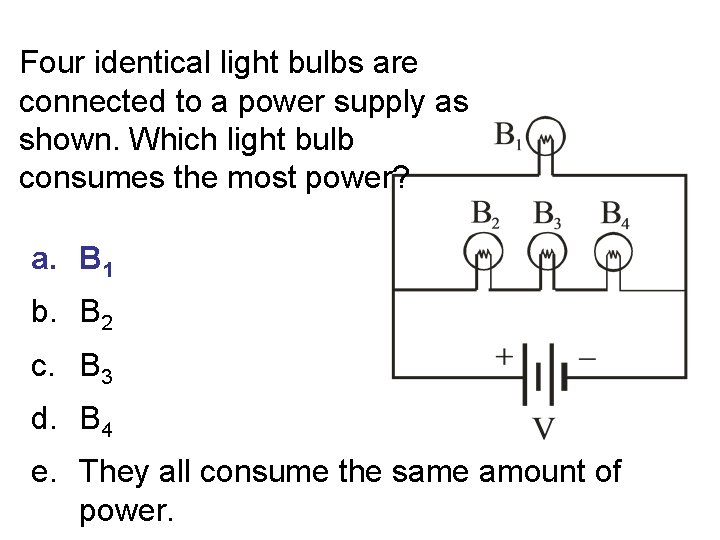Four identical light bulbs are connected to a power supply as shown. Which light bulb consumes the most power? a. B 1 b. B 2 c. B 3 d. B 4 e. They all consume the same amount of power.If two elements of a circuit are in parallel, they must have the same a. charge. b. potential difference across them. c. resistance. d. potential difference across them and the same current. e. current.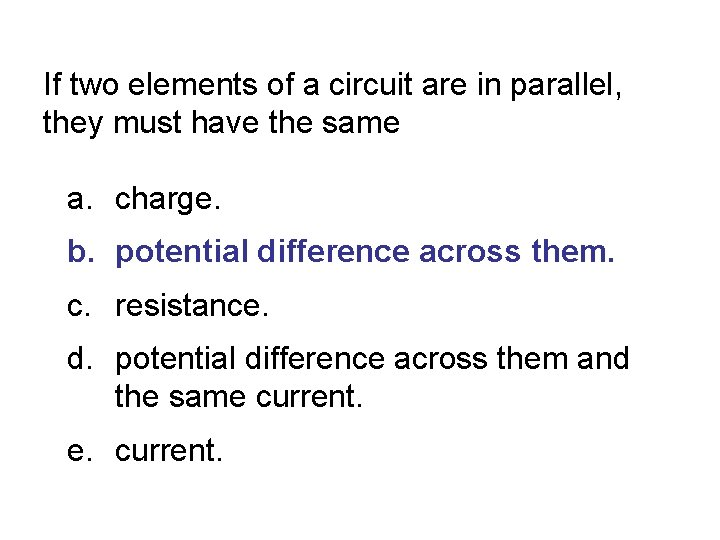If two elements of a circuit are in parallel, they must have the same a. charge. b. potential difference across them. c. resistance. d. potential difference across them and the same current. e. current.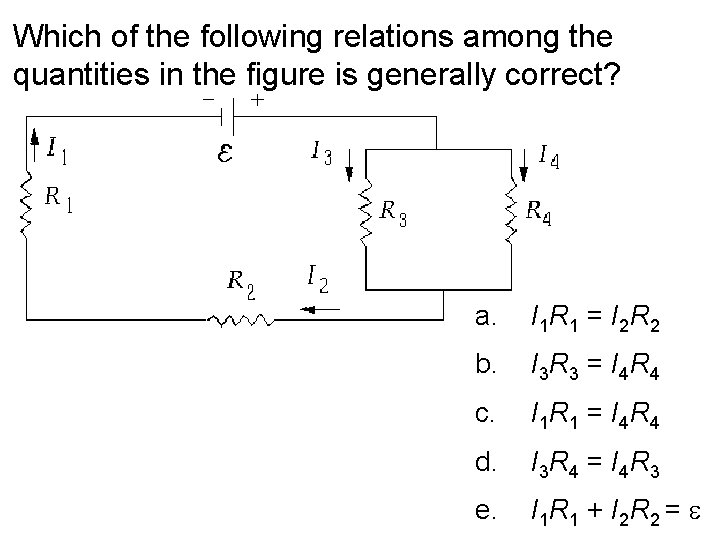Which of the following relations among the quantities in the figure is generally correct? a. I 1 R 1 = I 2 R 2 b. I 3 R 3 = I 4 R 4 c. I 1 R 1 = I 4 R 4 d. I 3 R 4 = I 4 R 3 e. I 1 R 1 + I 2 R 2 = e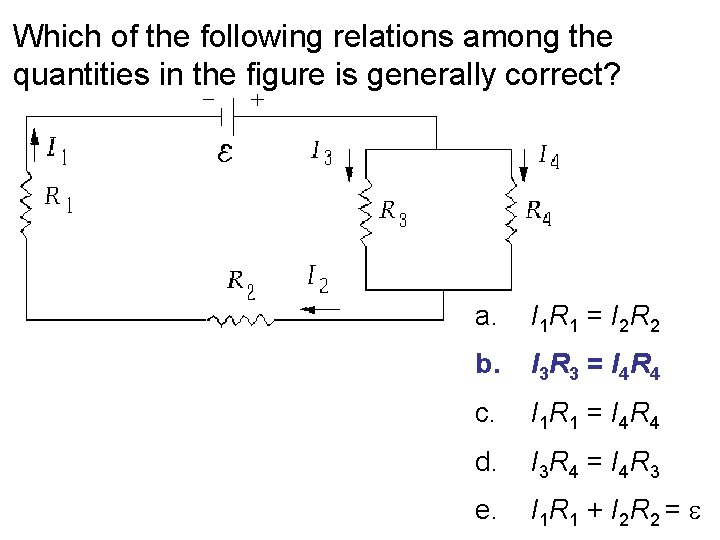Which of the following relations among the quantities in the figure is generally correct? a. I 1 R 1 = I 2 R 2 b. I 3 R 3 = I 4 R 4 c. I 1 R 1 = I 4 R 4 d. I 3 R 4 = I 4 R 3 e. I 1 R 1 + I 2 R 2 = eThree resistors are placed in a simple circuit. In which of the various configurations shown do all three resistors carry the same current?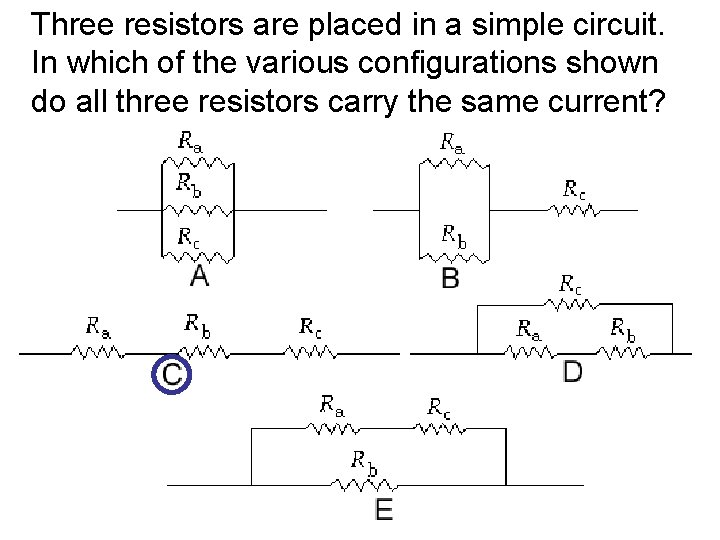Three resistors are placed in a simple circuit. In which of the various configurations shown do all three resistors carry the same current?The power delivered by the battery in the circuit shown is a. 2. 5 W b. 7. 0 W c. 3. 1 W d. 9. 7 W e. 5. 3 WThe power delivered by the battery in the circuit shown is a. 2. 5 W b. 7. 0 W c. 3. 1 W d. 9. 7 W e. 5. 3 W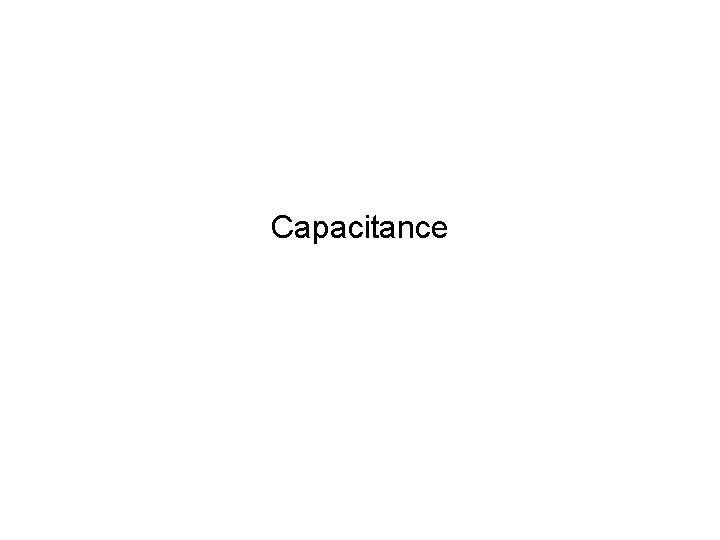CapacitanceA capacitor of capacitance C holds a charge Q when the potential difference across the plates is V. If the charge Q on the plates is doubled to 2 Q, a. the capacitance becomes (1/2)V. b. the capacitance becomes 2 C. c. the potential changes to (1/2)V. d. the potential changes to 2 V.A capacitor of capacitance C holds a charge Q when the potential difference across the plates is V. If the charge Q on the plates is doubled to 2 Q, a. the capacitance becomes (1/2)V. b. the capacitance becomes 2 C. c. the potential changes to (1/2)V. d. the potential changes to 2 V.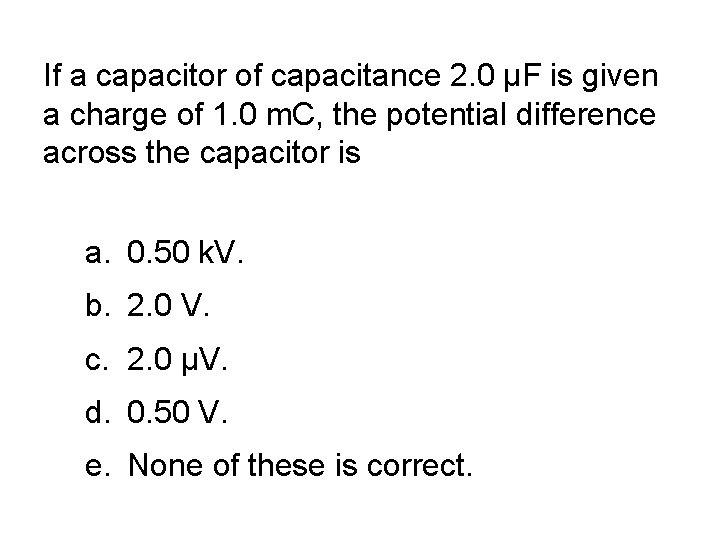If a capacitor of capacitance 2. 0 µF is given a charge of 1. 0 m. C, the potential difference across the capacitor is a. 0. 50 k. V. b. 2. 0 V. c. 2. 0 µV. d. 0. 50 V. e. None of these is correct.If a capacitor of capacitance 2. 0 µF is given a charge of 1. 0 m. C, the potential difference across the capacitor is a. 0. 50 k. V. b. 2. 0 V. c. 2. 0 µV. d. 0. 50 V. e. None of these is correct.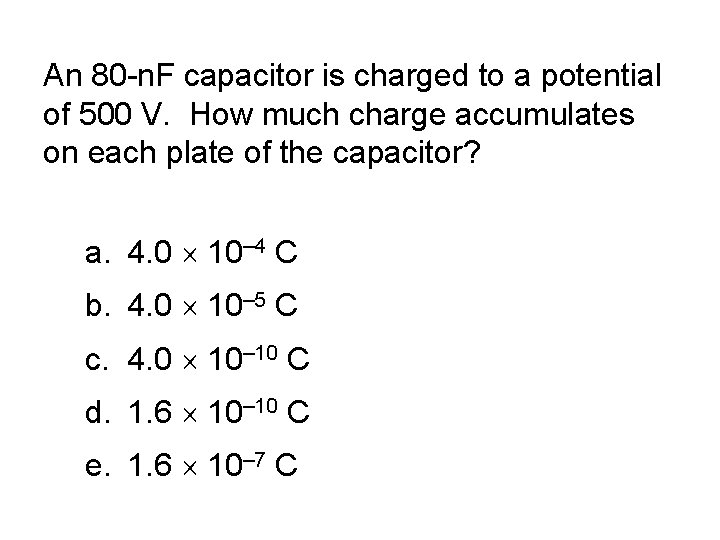An 80 -n. F capacitor is charged to a potential of 500 V. How much charge accumulates on each plate of the capacitor? a. 4. 0 ´ 10– 4 C b. 4. 0 ´ 10– 5 C c. 4. 0 ´ 10– 10 C d. 1. 6 ´ 10– 10 C e. 1. 6 ´ 10– 7 C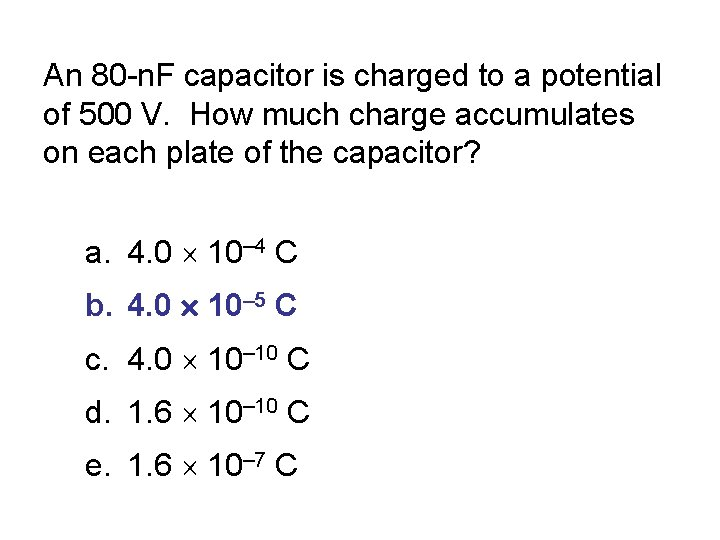An 80 -n. F capacitor is charged to a potential of 500 V. How much charge accumulates on each plate of the capacitor? a. 4. 0 ´ 10– 4 C b. 4. 0 ´ 10– 5 C c. 4. 0 ´ 10– 10 C d. 1. 6 ´ 10– 10 C e. 1. 6 ´ 10– 7 CDoubling the potential difference across a capacitor a. doubles its capacitance. b. halves its capacitance. c. quadruples the charge stored on the capacitor. d. halves the charge stored on the capacitor. e. does not change the capacitance of the capacitor.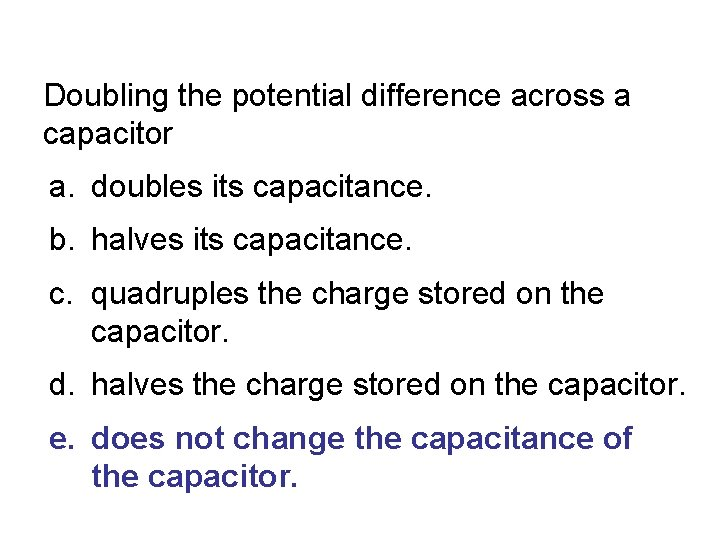Doubling the potential difference across a capacitor a. doubles its capacitance. b. halves its capacitance. c. quadruples the charge stored on the capacitor. d. halves the charge stored on the capacitor. e. does not change the capacitance of the capacitor.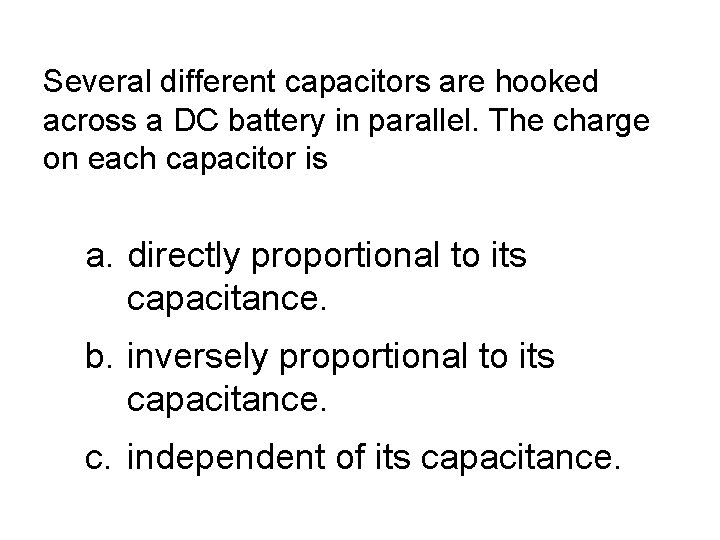Several different capacitors are hooked across a DC battery in parallel. The charge on each capacitor is a. directly proportional to its capacitance. b. inversely proportional to its capacitance. c. independent of its capacitance.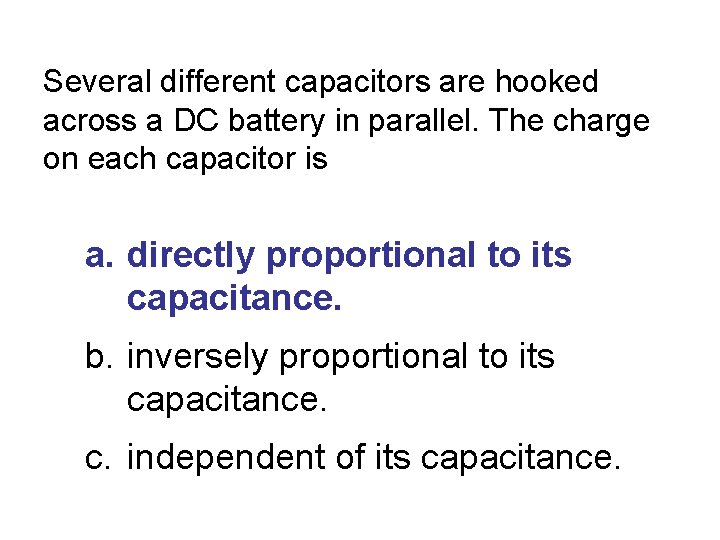Several different capacitors are hooked across a DC battery in parallel. The charge on each capacitor is a. directly proportional to its capacitance. b. inversely proportional to its capacitance. c. independent of its capacitance.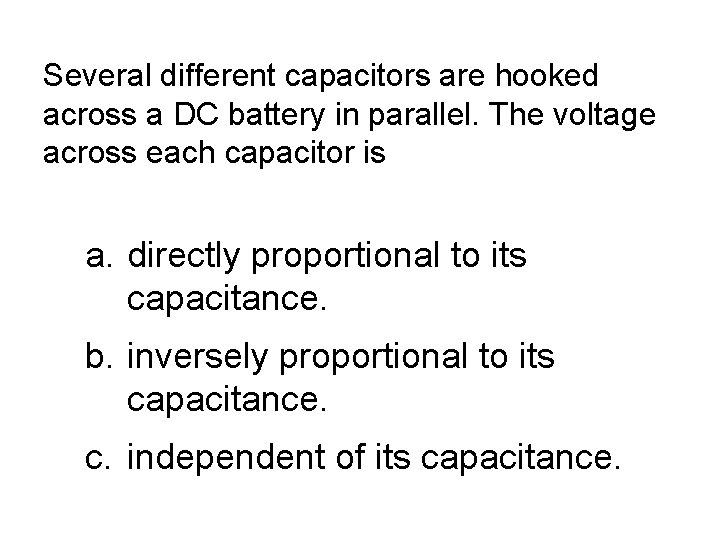Several different capacitors are hooked across a DC battery in parallel. The voltage across each capacitor is a. directly proportional to its capacitance. b. inversely proportional to its capacitance. c. independent of its capacitance.Several different capacitors are hooked across a DC battery in parallel. The voltage across each capacitor is a. directly proportional to its capacitance. b. inversely proportional to its capacitance. c. independent of its capacitance.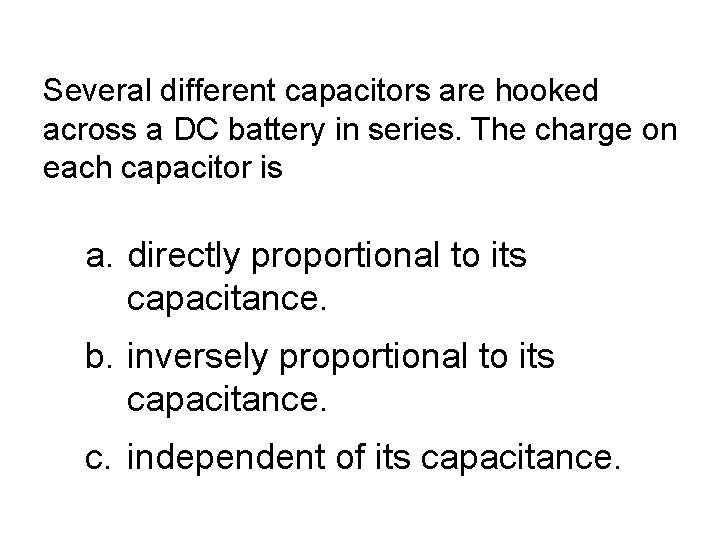Several different capacitors are hooked across a DC battery in series. The charge on each capacitor is a. directly proportional to its capacitance. b. inversely proportional to its capacitance. c. independent of its capacitance.Several different capacitors are hooked across a DC battery in series. The charge on each capacitor is a. directly proportional to its capacitance. b. inversely proportional to its capacitance. c. independent of its capacitance.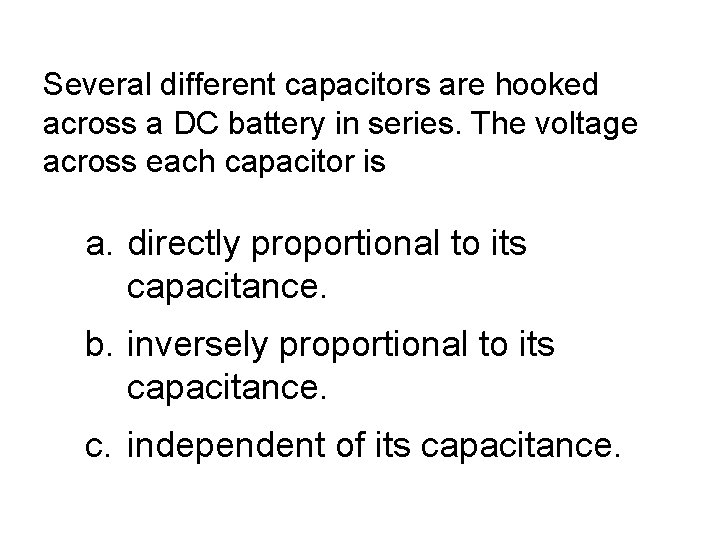Several different capacitors are hooked across a DC battery in series. The voltage across each capacitor is a. directly proportional to its capacitance. b. inversely proportional to its capacitance. c. independent of its capacitance.Several different capacitors are hooked across a DC battery in series. The voltage across each capacitor is a. directly proportional to its capacitance. b. inversely proportional to its capacitance. c. independent of its capacitance.